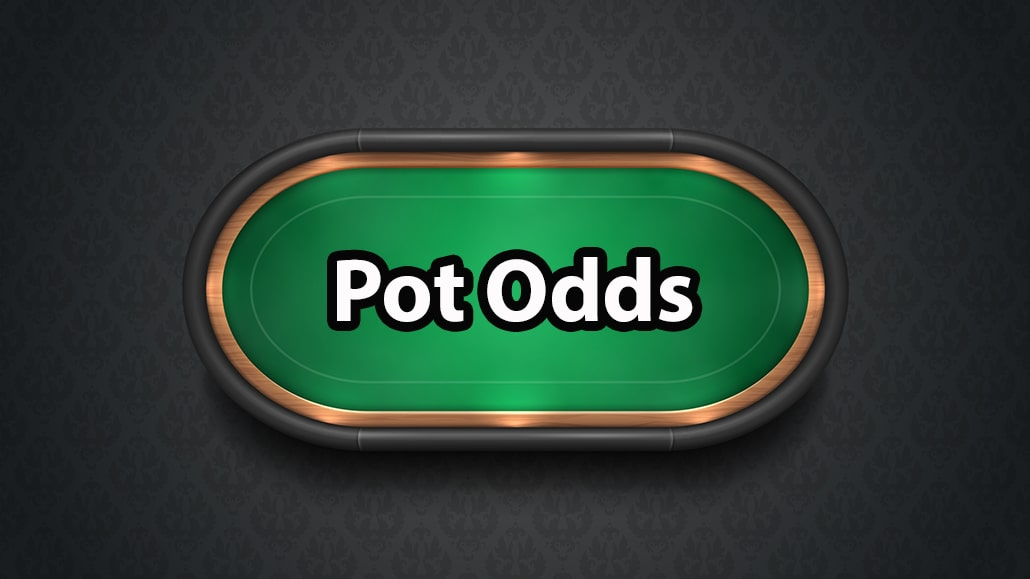# What Is Pot Odds In Poker?In poker, the term “Pot odds” refers to the ratio of the current size of the pot and the cost of calling a bet or a raise.

Pot odds are one of the most important concepts in poker, as it allows you to assess whether calling or folding will be the more profitable play in the long run.

The formula for calculating pot odds is:

The Amount you need to call / (The size of the current pot + The amount you need to call) x 100

To assess whether you should call after calculating the pot odds, you need to calculate how much equity your hand has against your opponent's range.

If your equity (chances of winning the hand) is higher than the pot odds you are getting, your call will be profitable in the long run.

## Poker Pot Odds Example:

Let’s say you are holding the nut flush draw on the flop, the pot is \$500, and your opponent goes all in for an additional \$500.

The first thing that you need to do is calculate the pot odds you are getting.

500 (the amount you need to call / 1000 (the current size of the pot) + 500 (the amount you need to call) x 100%

(500 / 1500) x 100 =

0.33 x 100 =

33%

In this situation, your hand needs at least 33% equity for your call to be profitable in the long run, or in other words, your hand needs to win more than 33% of the time for the call to be profitable.

Now let’s calculate how much equity your hand has.

In this situation, we can use the rule of 2 and 4. This rule states that to calculate how much equity your hand has, you must multiply your outs by 4 if there are two cards to come and 2 if there is one card to come.

For the sake of the example, let’s say that you need to hit your flush to win the hand and have 9 outs. That means that there are 9 cards in the deck that will help you make your hand.

Since we are on the flop, we need to multiply the number of outs by 4:

9 x 4 = 36

So, our hand has 36% equity in this situation, and since the chances that our hand will win are higher than the pot odds we are getting (33%), your call would be profitable in the long run.

ONLINE POKER:

RESOURCES: